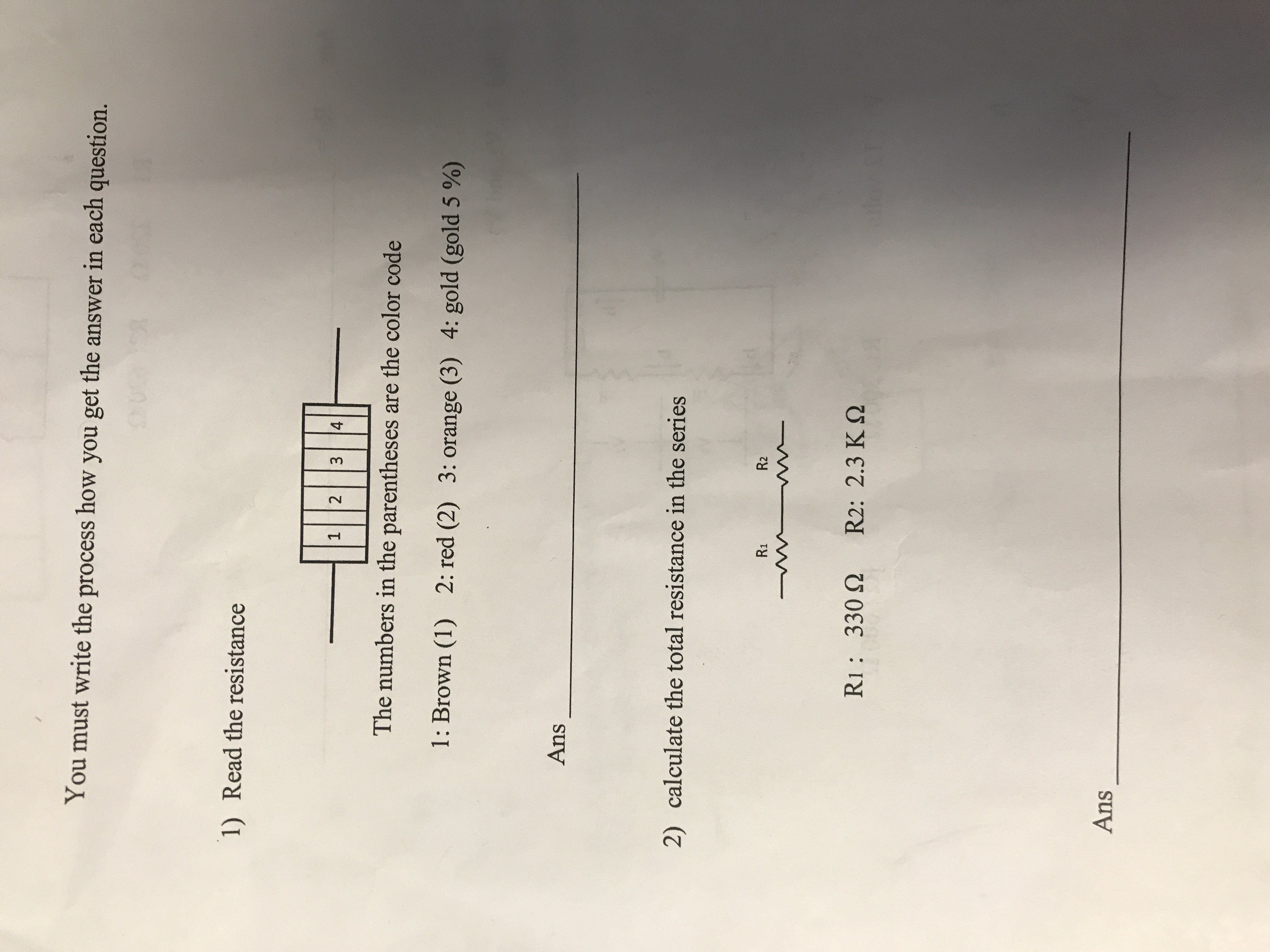You must write the process how you get the answer in each question.1)Read the resistance1 2 3 4The numbers in the parentheses are the color code1: Brown (1)2: red (2)3: orange (3)4: gold (gold 5 %)Ans2)calculate the total resistance in the seriesR1R2Ri: 3302 R2: 2.3 K2Ans

Question

1 & 2help_outlineImage TranscriptioncloseYou must write the process how you get the answer in each question. 1) Read the resistance 1 2 3 4 The numbers in the parentheses are the color code 1: Brown (1) 2: red (2) 3: orange (3) 4: gold (gold 5 %) Ans 2) calculate the total resistance in the series R1 R2 Ri: 3302 R2: 2.3 K2 Ans fullscreen
Step 1

(1)

In four band color code of resistors, the first band gives the first significant figure of the component value, the second band gives the second significant figure of the component value, the third band gives the number of trailing zeroes in the component value and the fourth band gives tolerance value in percent. Color black represents 0, brown corresponds to 1, red corresponds to 2, orange corresponds to 3, yellow corresponds to 4, green corresponds to 5, blue corresponds to 6, violet corresponds to 7, grey corresponds to 8 and white corresponds to 9. For the given resistor, the first band is brown corresponding to 1, the second band is red corresponding to 2 and the third band is orange corresponding to three zeroes. This implies the resistance is 12000 ohms with tolerance 5%.

Step 2

(2)The net resistance when the two resistors...

Want to see the full answer?

See Solution

Want to see this answer and more?

Our solutions are written by experts, many with advanced degrees, and available 24/7

See Solution
Tagged in

Physics